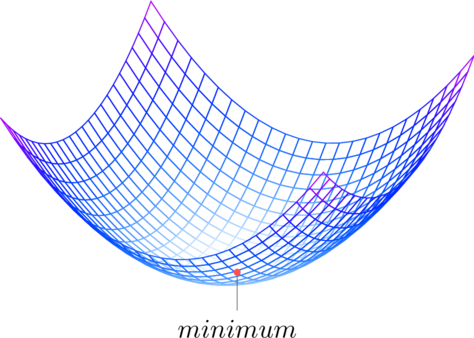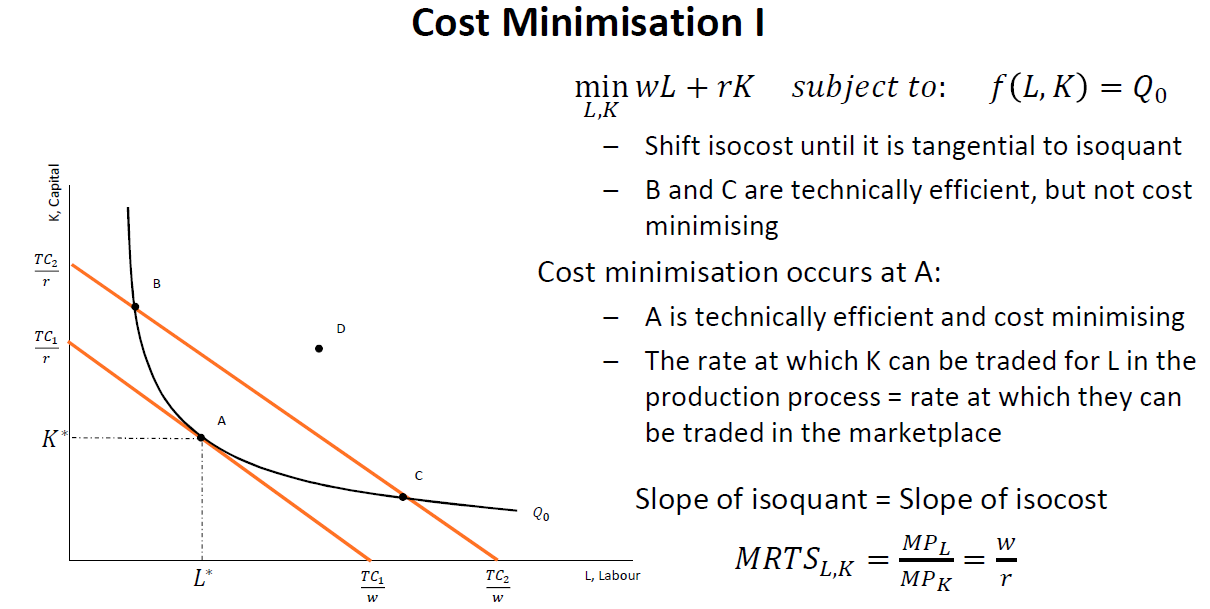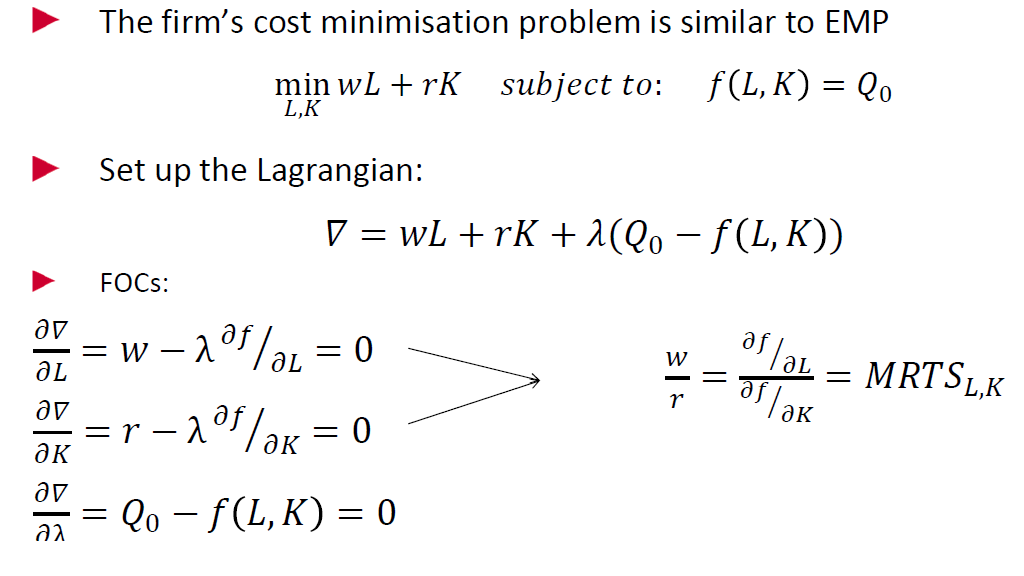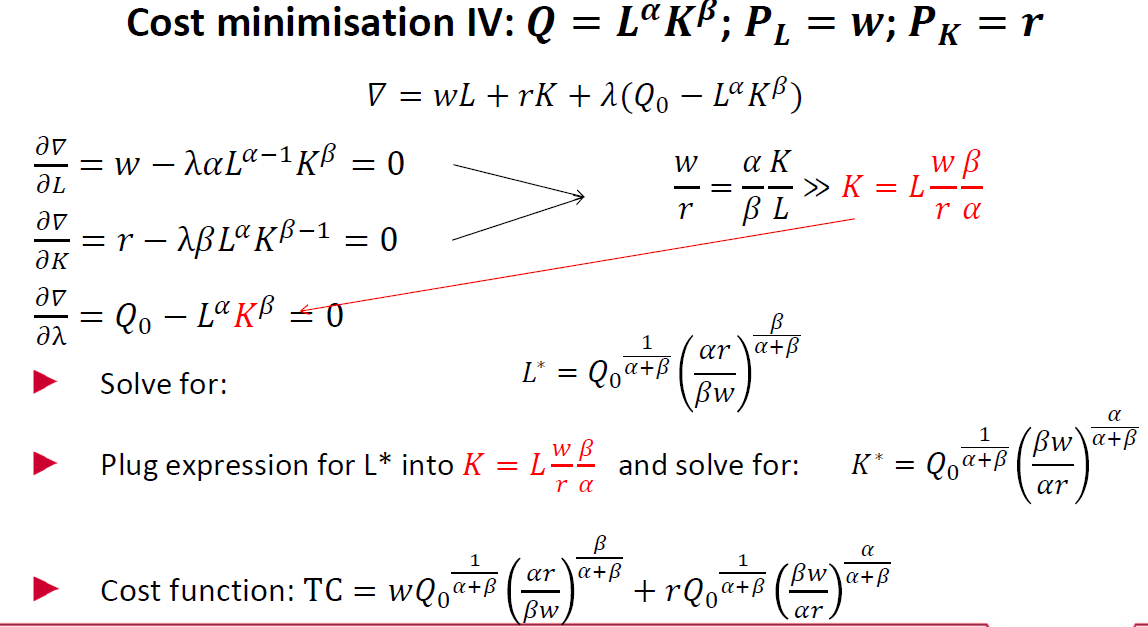# Cost Minimization – Intermediate Microeconomics Tutor New York London

## Intermediate Microeconomics tutor in NYC & LondonWork with our Intermediate Microeconomics Tutor in New York, London, or online. Our tutors are hired from NYU, Columbia, Yale, Princeton, LSE, Kings, UCL, Oxford, Warwick.

### Cost Minimization

Firms have a cost that depends on cost of factors of input. The two basic factors of production are labor and capital. Labor charges a wage rate and capital charges an interest rate. The total cost a firm pays is wage x labor + rent x capital. Cost minimization happens where the isoquant is tangent to the isocost curve. An Isocosts shows all the combinations of labour and capital that a producer could afford to purchase at a given set of input prices (w, r) and a total allowable cost level TC. Mathematically, cost minimization happens when their slopes are equal.### Optimization Problem

We set up a Lagrange with a target production level using a simple production function and a cost constraint. We take three partial derivatives with respect to labor, capital, and lambda. The Lagrange multiplier, λ, shows how much the optimal value of the objective function will change following a change in the constraint– By how much will costs increase when output constraint is increased marginally.Other popular courses:

Get Started

## See the #1 economics mentoring platform in action

Request a demo